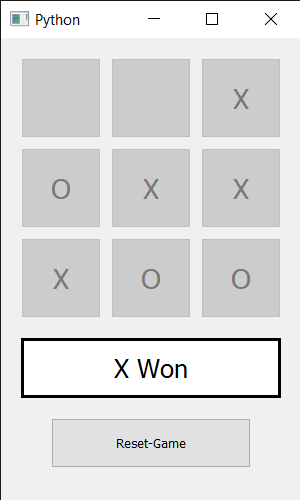# Tic Tac Toe Game using PyQt5 in Python

• Last Updated : 01 Aug, 2020

In this article , we will see how we can create a Tic Tac Toe game using PyQt5. Tic-tac-toe, noughts, and crosses, or Xs and Os is a paper-and-pencil game for two players, X and O, who take turns marking the spaces in a 3×3 grid. The player who succeeds in placing three of their marks in a horizontal, vertical, or diagonal row is the winner.

Below is how the Tic Tac Toe game will look like :GUI implementation steps :
1. Create a list of push buttons
2. Arrange them in 3×3 order and add font to them
3. Create a label below the push buttons that tells the result
4. Set alignment and font to the label
5. Add another push button for reset the game at the bottom

Back end implementation steps :
1. Create two variable to know whose chance is and to know how many chances are completed
2. Add same action to the list of buttons such that each buttons should call the same action
3. Inside the action method get the button by whom method is called using sender method
4. Set the text to the button according to the chance and make it disabled so that it can’t be pressed again and call the who_wins method
5. Set text according to the answer returned by the who_wins method
6. Inside the who_wins method check if rows, columns and diagonals are crossed or not.
7. If someone won the match set the text to the label and make all the buttons disabled
8. Add action to the reset button
9. Inside the reset button action make the variable value set to the starting value and make all the push button enabled and make the label and button text to blank.

Below is the implementation :

## Python3

 `# importing required libraries``from` `PyQt5.QtWidgets ``import` `*``from` `PyQt5 ``import` `QtCore, QtGui``from` `PyQt5.QtGui ``import` `*``from` `PyQt5.QtCore ``import` `*`` ` `import` `sys`` ` `# create a Window class``class` `Window(QMainWindow):``    ``# constructor``    ``def` `__init__(``self``):``        ``super``().__init__()`` ` `        ``# setting title``        ``self``.setWindowTitle(``"Python "``)`` ` `        ``# setting geometry``        ``self``.setGeometry(``100``, ``100``, ``                         ``300``, ``500``)`` ` `        ``# calling method``        ``self``.UiComponents()`` ` `        ``# showing all the widgets``        ``self``.show()`` ` `    ``# method for components``    ``def` `UiComponents(``self``):`` ` `        ``# turn``        ``self``.turn ``=` `0`` ` `        ``# times``        ``self``.times ``=` `0`` ` `        ``# creating a push button list``        ``self``.push_list ``=` `[]`` ` `        ``# creating 2d list``        ``for` `_ ``in` `range``(``3``):``            ``temp ``=` `[]``            ``for` `_ ``in` `range``(``3``):``                ``temp.append((QPushButton(``self``)))``            ``# adding 3 push button in single row``            ``self``.push_list.append(temp)`` ` `        ``# x and y co-ordinate``        ``x ``=` `90``        ``y ``=` `90`` ` `        ``# traversing through push button list``        ``for` `i ``in` `range``(``3``):``            ``for` `j ``in` `range``(``3``):`` ` `                ``# setting geometry to the button``                ``self``.push_list[i][j].setGeometry(x``*``i ``+` `20``, ``                                                 ``y``*``j ``+` `20``,``                                                 ``80``, ``80``)`` ` `                ``# setting font to the button``                ``self``.push_list[i][j].setFont(QFont(QFont(``'Times'``, ``17``)))`` ` `                ``# adding action``                ``self``.push_list[i][j].clicked.connect(``self``.action_called)`` ` `        ``# creating label to tel the score``        ``self``.label ``=` `QLabel(``self``)`` ` `        ``# setting geometry to the label``        ``self``.label.setGeometry(``20``, ``300``, ``260``, ``60``)`` ` `        ``# setting style sheet to the label``        ``self``.label.setStyleSheet(``"QLabel"``                                 ``"{"``                                 ``"border : 3px solid black;"``                                 ``"background : white;"``                                 ``"}"``)`` ` `        ``# setting label alignment``        ``self``.label.setAlignment(Qt.AlignCenter)`` ` `        ``# setting font to the label``        ``self``.label.setFont(QFont(``'Times'``, ``15``))`` ` `        ``# creating push button to restart the score``        ``reset_game ``=` `QPushButton(``"Reset-Game"``, ``self``)`` ` `        ``# setting geometry``        ``reset_game.setGeometry(``50``, ``380``, ``200``, ``50``)`` ` `        ``# adding action action to the reset push button``        ``reset_game.clicked.connect(``self``.reset_game_action)`` ` ` ` `    ``# method called by reset button``    ``def` `reset_game_action(``self``):`` ` `        ``# resetting values``        ``self``.turn ``=` `0``        ``self``.times ``=` `0`` ` `        ``# making label text empty:``        ``self``.label.setText("")`` ` `        ``# traversing push list``        ``for` `buttons ``in` `self``.push_list:``            ``for` `button ``in` `buttons:``                ``# making all the button enabled``                ``button.setEnabled(``True``)``                ``# removing text of all the buttons``                ``button.setText("")`` ` `    ``# action called by the push buttons``    ``def` `action_called(``self``):`` ` `        ``self``.times ``+``=` `1`` ` `        ``# getting button which called the action``        ``button ``=` `self``.sender()`` ` `        ``# making button disabled``        ``button.setEnabled(``False``)`` ` `        ``# checking the turn``        ``if` `self``.turn ``=``=` `0``:``            ``button.setText(``"X"``)``            ``self``.turn ``=` `1``        ``else``:``            ``button.setText(``"O"``)``            ``self``.turn ``=` `0`` ` `        ``# call the winner checker method``        ``win ``=` `self``.who_wins()``         ` `        ``# text``        ``text ``=` `""`` ` `        ``# if winner is decided``        ``if` `win ``=``=` `True``:``            ``# if current chance is 0``            ``if` `self``.turn ``=``=` `0``:``                ``# O has won``                ``text ``=` `"O Won"``            ``# X has won``            ``else``:``                ``text ``=` `"X Won"`` ` `            ``# disabling all the buttons``            ``for` `buttons ``in` `self``.push_list:``                ``for` `push ``in` `buttons:``                    ``push.setEnabled(``False``)`` ` `        ``# if winner is not decided``        ``# and total times is 9``        ``elif` `self``.times ``=``=` `9``:``            ``text ``=` `"Match is Draw"`` ` `        ``# setting text to the label``        ``self``.label.setText(text)`` ` ` ` `    ``# method to check who wins``    ``def` `who_wins(``self``):`` ` `        ``# checking if any row crossed``        ``for` `i ``in` `range``(``3``):``            ``if` `self``.push_list[``0``][i].text() ``=``=` `self``.push_list[``1``][i].text() \``                    ``and` `self``.push_list[``0``][i].text() ``=``=` `self``.push_list[``2``][i].text() \``                    ``and` `self``.push_list[``0``][i].text() !``=` `"":``                ``return` `True`` ` `        ``# checking if any column crossed``        ``for` `i ``in` `range``(``3``):``            ``if` `self``.push_list[i][``0``].text() ``=``=` `self``.push_list[i][``1``].text() \``                    ``and` `self``.push_list[i][``0``].text() ``=``=` `self``.push_list[i][``2``].text() \``                    ``and` `self``.push_list[i][``0``].text() !``=` `"":``                ``return` `True`` ` `        ``# checking if diagonal crossed``        ``if` `self``.push_list[``0``][``0``].text() ``=``=` `self``.push_list[``1``][``1``].text() \``                ``and` `self``.push_list[``0``][``0``].text() ``=``=` `self``.push_list[``2``][``2``].text() \``                ``and` `self``.push_list[``0``][``0``].text() !``=` `"":``            ``return` `True`` ` `        ``# if other diagonal is crossed``        ``if` `self``.push_list[``0``][``2``].text() ``=``=` `self``.push_list[``1``][``1``].text() \``                ``and` `self``.push_list[``1``][``1``].text() ``=``=` `self``.push_list[``2``][``0``].text() \``                ``and` `self``.push_list[``0``][``2``].text() !``=` `"":``            ``return` `True`` ` ` ` `        ``#if nothing is crossed``        ``return` `False`` ` ` ` ` ` `# create pyqt5 app``App ``=` `QApplication(sys.argv)`` ` `# create the instance of our Window``window ``=` `Window()`` ` `# start the app``sys.exit(App.``exec``())`

Output :

My Personal Notes arrow_drop_up